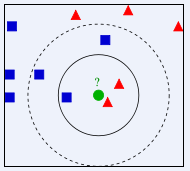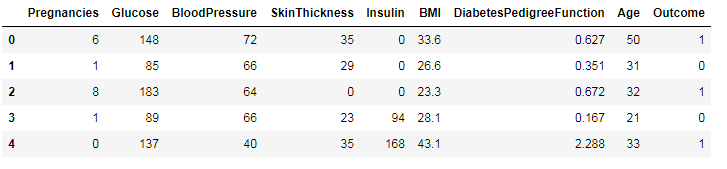#sklearn调包侠之KNN算法

0
0
01. 云栖社区>
2. 博客>
3. 正文

## sklearn调包侠之KNN算法tips：在本篇中会按小抄详细过一遍，之后就可能会随意一些。

### KNN算法原理KNN算法原理

### 实战——糖尿病预测

##### 数据导入

（链接：https://pan.baidu.com/s/1gqaGuQ9kWZFfc-SXbYFDkA 密码：lxfx）

``````import numpy as np
import pandas as pd

``````• Pregnancies：怀孕的次数
• Glucose：血浆葡萄糖浓度
• BloodPressure：舒张压
• SkinThickness：肱三头肌皮肤皱皱厚度
• Insulin： 胰岛素
• BMI：身体质量指数
• Dia....：糖尿病血统指数
• Age：年龄
• Outcone：是否糖尿病，1为是

``````X = data.iloc[:, 0:8]
Y = data.iloc[:, 8]
``````
##### 切分数据集

``````from sklearn.model_selection import train_test_split
X_train, X_test, Y_train, Y_test = train_test_split(X, Y, test_size=0.2, random_state=22)
``````

##### 模型训练与评估

KNN算法使用sklearn.neighbors模块中的KNeighborsClassifier方法。常用的参数如下：

• n_neighbors，整数，也就是k值。
• weights，默认为‘uniform’；这个参数可以针对不同的邻居指定不同的权重，也就是说，越近可以权重越高，默认是一样的权重。‘distance’可以设置不同权重。

``````from sklearn.neighbors import KNeighborsClassifier,RadiusNeighborsClassifier

model1 = KNeighborsClassifier(n_neighbors=2)
model1.fit(X_train, Y_train)
score1 = model1.score(X_test, Y_test)

model2 = KNeighborsClassifier(n_neighbors=2, weights='distance')
model2.fit(X_train, Y_train)
score2 = model2.score(X_test, Y_test)

model3.fit(X_train, Y_train)
score3 = model3.score(X_test, Y_test)

print(score1, score2, score3)

#result
#0.714285714286 0.701298701299 0.649350649351
``````

##### 交叉验证

``````from sklearn.model_selection import cross_val_score

result1 = cross_val_score(model1, X, Y, cv=10)
result2 = cross_val_score(model2, X, Y, cv=10)
result3 = cross_val_score(model3, X, Y, cv=10)

print(result1.mean(), result2.mean(), result3.mean())

# result
# 0.712235133288 0.67966507177 0.64976076555
``````

+ 关注